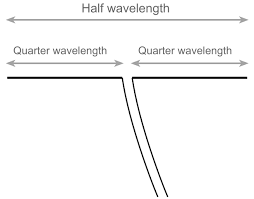### Notes of Electromagnetics [EX 503]

#### Antennas

Introduction to Antenna

Antenna

- Antenna is a structure made of metallic object like wires, used to convert high frequency current into electromagnetic wave and vice versa.
- It can acts as both transmitter and receiver.
- It radiates electromagnetic energy into space and also accumulates electromagnetic energy from space.
- A good antenna design improves performance of communication system.
- The basic principle of antenna are:
a) The radiation is produced by accelerated or decelerated charge.
b) I L = Q v

Parameters or Properties of Antenna

1. Antenna Pattern:
- It is the 3 Dimensional plot of its radiation.
- If amplitude of component of E vector is plotted, field or voltage pattern is obtained.
- The square of amplitude of E vector when plotted, gives power pattern.

- It indicates the strength of electromagnetic wave radiation.
- It is given as:
U = r^2 * P(ave)
where, P(ave) = total average power radiated.

3. Directive Gain [Gd(θ, ϕ)]
- It is the measure of concentration of radiated power in particular direction.
- It is the ability to direct radiated power in given direction.
- The general equation is:
(Max directive gain / Directivity(D)) = (Max. radiation intensity / Average radiation intensity)

4. Power Gain [Gp(θ, ϕ)]
- It is the ratio of radiation intensity to the total input power to antenna multiplied by 4π.
- The general equation is:
Gp = 4 π * { U(θ, ϕ) / P(in) }
where,
P(in) = P(i) + P(rad) ; P(i) is ohmic power loss and P(rad) is power radiated by antenna.
So, P(in) = 1/2 * [ I(m) ^ 2 * { R(i) + R(rad) } ]

- The radiation efficiency is defined as the ratio of power gain to directive gain.
- It is given as:
Efficiency = Gp / Gd = P(rad) / P(in) = R(rad) / { R(rad) + R(i) }

Antenna Types and Properties

Types of Antenna

1. Wire Antenna:
- It is the most commonly used antenna type.
- It may be straight wire (dipole) antenna, loop antenna or helix antenna.

2. Aperture Antenna:
- It is used in aircraft and spacecraft.
- It can be covered with dielectric for environmental protection.
- It can be flush mounted on skin of aircraft.

3. Microstrip Antenna:
- It is used for governmental and commercial applications.
- It has metallic patch on grounded substrate.
- It has low profile.
- It is comfortable, simple and inexpensive.

4. Array Antenna:
- It is used to obtain radiation characteristics by different arrangement of radiating elements.

5. Reflector Antenna:
- It is used for very long distance communication.

6. Lens Antenna:
- It is used to collimate incident divergent energy so as to prevent it from spreading in undesired direction.